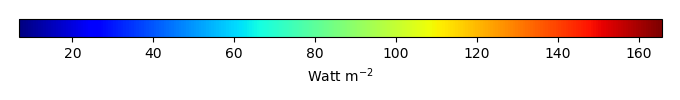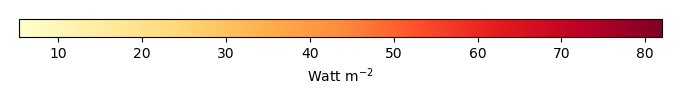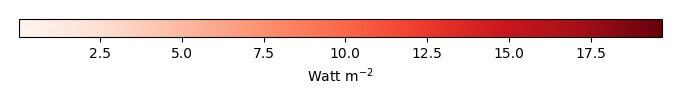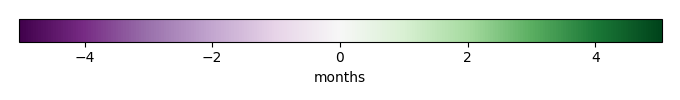# Mean State

Period Mean (original grids) [Watt m-2]
Model Period Mean (intersection) [Watt m-2]
Model Period Mean (complement) [Watt m-2]
Benchmark Period Mean (intersection) [Watt m-2]
Benchmark Period Mean (complement) [Watt m-2]
Bias [Watt m-2]
RMSE [Watt m-2]
Phase Shift [months]
Bias Score 
RMSE Score 
Seasonal Cycle Score 
Spatial Distribution Score 
Interannual Variability Score 
Overall Score 
Benchmark [-] 38.3
CLM4 [-] 36.8 36.5 0.00 39.6 15.5 -2.56 10.9 1.71 0.37 0.37 0.72 0.91 0.47 0.54
CLM4.5 [-] 36.4 36.1 0.00 39.6 15.5 -2.83 11.1 1.88 0.36 0.37 0.69 0.92 0.47 0.53
CLM5 [-] 36.3 36.0 0.00 39.6 15.5 -3.01 10.6 1.70 0.38 0.37 0.72 0.95 0.48 0.55
Period Mean (original grids) [Watt m-2]
Model Period Mean (intersection) [Watt m-2]
Model Period Mean (complement) [Watt m-2]
Benchmark Period Mean (intersection) [Watt m-2]
Benchmark Period Mean (complement) [Watt m-2]
Bias [Watt m-2]
RMSE [Watt m-2]
Phase Shift [months]
Bias Score 
RMSE Score 
Seasonal Cycle Score 
Spatial Distribution Score 
Interannual Variability Score 
Overall Score 
Benchmark [-] 52.3
CLM4 [-] 48.7 49.2 0.00 52.8 14.1 -3.75 12.9 1.25 0.48 0.38 0.84 0.99 0.55 0.60
CLM4.5 [-] 47.7 48.2 0.00 52.8 14.1 -4.69 13.3 1.18 0.45 0.38 0.85 0.99 0.55 0.60
CLM5 [-] 47.2 47.6 0.00 52.8 14.1 -5.21 13.3 1.35 0.44 0.39 0.83 0.99 0.53 0.60
Period Mean (original grids) [Watt m-2]
Model Period Mean (intersection) [Watt m-2]
Model Period Mean (complement) [Watt m-2]
Benchmark Period Mean (intersection) [Watt m-2]
Benchmark Period Mean (complement) [Watt m-2]
Bias [Watt m-2]
RMSE [Watt m-2]
Phase Shift [months]
Bias Score 
RMSE Score 
Seasonal Cycle Score 
Spatial Distribution Score 
Interannual Variability Score 
Overall Score 
Benchmark [-] 39.3
CLM4 [-] 37.4 37.4 0.00 40.4 16.6 -2.81 11.9 1.86 0.42 0.40 0.71 0.93 0.56 0.57
CLM4.5 [-] 36.8 36.8 0.00 40.4 16.6 -3.31 12.3 1.83 0.40 0.39 0.72 0.94 0.57 0.57
CLM5 [-] 36.9 36.9 0.00 40.4 16.6 -3.30 11.9 1.76 0.41 0.41 0.73 0.91 0.56 0.57
Period Mean (original grids) [Watt m-2]
Model Period Mean (intersection) [Watt m-2]
Model Period Mean (complement) [Watt m-2]
Benchmark Period Mean (intersection) [Watt m-2]
Benchmark Period Mean (complement) [Watt m-2]
Bias [Watt m-2]
RMSE [Watt m-2]
Phase Shift [months]
Bias Score 
RMSE Score 
Seasonal Cycle Score 
Spatial Distribution Score 
Interannual Variability Score 
Overall Score 
Benchmark [-] 31.6
CLM4 [-] 45.4 45.3 0.00 31.5 32.6 14.0 28.2 0.236 0.62 0.48 0.98 0.98 0.77 0.72
CLM4.5 [-] 39.3 39.2 0.00 31.5 32.6 9.07 22.5 0.241 0.73 0.55 0.98 0.90 0.72 0.74
CLM5 [-] 36.8 36.6 0.00 31.5 32.6 6.48 20.3 0.340 0.77 0.58 0.98 0.88 0.71 0.75
Period Mean (original grids) [Watt m-2]
Model Period Mean (intersection) [Watt m-2]
Model Period Mean (complement) [Watt m-2]
Benchmark Period Mean (intersection) [Watt m-2]
Benchmark Period Mean (complement) [Watt m-2]
Bias [Watt m-2]
RMSE [Watt m-2]
Phase Shift [months]
Bias Score 
RMSE Score 
Seasonal Cycle Score 
Spatial Distribution Score 
Interannual Variability Score 
Overall Score 
Benchmark [-] 23.5
CLM4 [-] 29.3 29.3 0.00 25.9 15.5 3.86 11.0 1.90 0.42 0.37 0.71 0.21 0.46 0.42
CLM4.5 [-] 29.3 29.3 0.00 25.9 15.5 3.86 11.0 1.89 0.42 0.37 0.70 0.23 0.45 0.42
CLM5 [-] 29.7 29.6 0.00 25.9 15.5 4.21 11.0 2.04 0.43 0.38 0.68 0.20 0.43 0.42
Period Mean (original grids) [Watt m-2]
Model Period Mean (intersection) [Watt m-2]
Model Period Mean (complement) [Watt m-2]
Benchmark Period Mean (intersection) [Watt m-2]
Benchmark Period Mean (complement) [Watt m-2]
Bias [Watt m-2]
RMSE [Watt m-2]
Phase Shift [months]
Bias Score 
RMSE Score 
Seasonal Cycle Score 
Spatial Distribution Score 
Interannual Variability Score 
Overall Score 
Benchmark [-] 21.4
CLM4 [-] 26.1 26.0 0.00 21.5 17.6 4.45 11.7 0.479 0.70 0.51 0.94 0.78 0.70 0.69
CLM4.5 [-] 28.0 27.9 0.00 21.5 17.6 6.30 13.8 0.515 0.65 0.47 0.95 0.70 0.68 0.65
CLM5 [-] 25.5 25.4 0.00 21.5 17.6 3.83 10.8 0.499 0.73 0.52 0.94 0.87 0.70 0.71
Period Mean (original grids) [Watt m-2]
Model Period Mean (intersection) [Watt m-2]
Model Period Mean (complement) [Watt m-2]
Benchmark Period Mean (intersection) [Watt m-2]
Benchmark Period Mean (complement) [Watt m-2]
Bias [Watt m-2]
RMSE [Watt m-2]
Phase Shift [months]
Bias Score 
RMSE Score 
Seasonal Cycle Score 
Spatial Distribution Score 
Interannual Variability Score 
Overall Score 
Benchmark [-] 30.0
CLM4 [-] 40.2 40.1 0.00 30.5 14.9 8.90 14.4 1.07 0.53 0.45 0.86 0.90 0.64 0.64
CLM4.5 [-] 40.9 40.9 0.00 30.5 14.9 9.57 14.9 1.07 0.50 0.44 0.86 0.90 0.65 0.63
CLM5 [-] 39.2 39.1 0.00 30.5 14.9 7.88 13.8 1.08 0.55 0.45 0.86 0.97 0.66 0.65
Period Mean (original grids) [Watt m-2]
Model Period Mean (intersection) [Watt m-2]
Model Period Mean (complement) [Watt m-2]
Benchmark Period Mean (intersection) [Watt m-2]
Benchmark Period Mean (complement) [Watt m-2]
Bias [Watt m-2]
RMSE [Watt m-2]
Phase Shift [months]
Bias Score 
RMSE Score 
Seasonal Cycle Score 
Spatial Distribution Score 
Interannual Variability Score 
Overall Score 
Benchmark [-] 29.5
CLM4 [-] 38.9 38.8 0.00 30.6 14.2 7.31 13.2 0.963 0.53 0.39 0.89 0.93 0.53 0.61
CLM4.5 [-] 38.2 38.2 0.00 30.6 14.2 6.70 13.1 0.893 0.51 0.39 0.90 0.94 0.51 0.61
CLM5 [-] 37.6 37.6 0.00 30.6 14.2 6.13 12.4 0.935 0.55 0.41 0.89 0.98 0.48 0.62
Period Mean (original grids) [Watt m-2]
Model Period Mean (intersection) [Watt m-2]
Model Period Mean (complement) [Watt m-2]
Benchmark Period Mean (intersection) [Watt m-2]
Benchmark Period Mean (complement) [Watt m-2]
Bias [Watt m-2]
RMSE [Watt m-2]
Phase Shift [months]
Bias Score 
RMSE Score 
Seasonal Cycle Score 
Spatial Distribution Score 
Interannual Variability Score 
Overall Score 
Benchmark [-] 33.0
CLM4 [-] 41.9 41.7 0.00 32.8 37.1 9.07 25.3 0.170 0.73 0.52 0.99 0.95 0.75 0.74
CLM4.5 [-] 40.1 39.9 0.00 32.8 37.1 7.69 24.6 0.295 0.75 0.54 0.98 0.98 0.68 0.75
CLM5 [-] 35.1 34.9 0.00 32.8 37.1 2.86 19.3 0.271 0.81 0.60 0.98 0.98 0.65 0.77
Period Mean (original grids) [Watt m-2]
Model Period Mean (intersection) [Watt m-2]
Model Period Mean (complement) [Watt m-2]
Benchmark Period Mean (intersection) [Watt m-2]
Benchmark Period Mean (complement) [Watt m-2]
Bias [Watt m-2]
RMSE [Watt m-2]
Phase Shift [months]
Bias Score 
RMSE Score 
Seasonal Cycle Score 
Spatial Distribution Score 
Interannual Variability Score 
Overall Score 
Benchmark [-] 35.1
CLM4 [-] 41.9 41.9 0.00 36.7 14.1 4.04 10.6 0.638 0.59 0.54 0.95 0.93 0.60 0.69
CLM4.5 [-] 41.8 41.8 0.00 36.7 14.1 3.97 10.5 0.650 0.59 0.54 0.95 0.95 0.60 0.69
CLM5 [-] 40.8 40.8 0.00 36.7 14.1 3.07 10.8 0.654 0.58 0.53 0.95 0.90 0.64 0.69
Period Mean (original grids) [Watt m-2]
Model Period Mean (intersection) [Watt m-2]
Model Period Mean (complement) [Watt m-2]
Benchmark Period Mean (intersection) [Watt m-2]
Benchmark Period Mean (complement) [Watt m-2]
Bias [Watt m-2]
RMSE [Watt m-2]
Phase Shift [months]
Bias Score 
RMSE Score 
Seasonal Cycle Score 
Spatial Distribution Score 
Interannual Variability Score 
Overall Score 
Benchmark [-] 19.8
CLM4 [-] 27.8 27.8 0.00 20.7 10.0 6.44 13.0 0.866 0.62 0.50 0.91 0.93 0.67 0.69
CLM4.5 [-] 26.9 26.9 0.00 20.7 10.0 5.62 11.8 1.08 0.62 0.51 0.87 0.97 0.69 0.69
CLM5 [-] 26.8 26.9 0.00 20.7 10.0 5.61 11.4 1.02 0.62 0.52 0.89 0.97 0.68 0.70
Period Mean (original grids) [Watt m-2]
Model Period Mean (intersection) [Watt m-2]
Model Period Mean (complement) [Watt m-2]
Benchmark Period Mean (intersection) [Watt m-2]
Benchmark Period Mean (complement) [Watt m-2]
Bias [Watt m-2]
RMSE [Watt m-2]
Phase Shift [months]
Bias Score 
RMSE Score 
Seasonal Cycle Score 
Spatial Distribution Score 
Interannual Variability Score 
Overall Score 
Benchmark [-] 23.6
CLM4 [-] 49.0 49.0 0.00 43.1 14.1 4.96 17.5 0.872 0.58 0.48 0.89 0.87 0.57 0.64
CLM4.5 [-] 48.4 48.4 0.00 43.1 14.1 4.45 17.0 0.908 0.57 0.48 0.88 0.85 0.56 0.64
CLM5 [-] 47.6 47.6 0.00 43.1 14.1 3.75 16.6 0.898 0.59 0.48 0.89 0.88 0.56 0.65
Period Mean (original grids) [Watt m-2]
Model Period Mean (intersection) [Watt m-2]
Model Period Mean (complement) [Watt m-2]
Benchmark Period Mean (intersection) [Watt m-2]
Benchmark Period Mean (complement) [Watt m-2]
Bias [Watt m-2]
RMSE [Watt m-2]
Phase Shift [months]
Bias Score 
RMSE Score 
Seasonal Cycle Score 
Spatial Distribution Score 
Interannual Variability Score 
Overall Score 
Benchmark [-] 64.3
CLM4 [-] 83.3 83.2 0.00 66.2 16.5 15.0 21.9 0.619 0.50 0.56 0.94 0.99 0.51 0.68
CLM4.5 [-] 82.7 82.5 0.00 66.2 16.5 14.3 21.5 0.633 0.51 0.56 0.94 0.99 0.51 0.68
CLM5 [-] 81.5 81.3 0.00 66.2 16.5 13.1 21.0 0.697 0.53 0.55 0.93 0.98 0.53 0.68
Period Mean (original grids) [Watt m-2]
Model Period Mean (intersection) [Watt m-2]
Model Period Mean (complement) [Watt m-2]
Benchmark Period Mean (intersection) [Watt m-2]
Benchmark Period Mean (complement) [Watt m-2]
Bias [Watt m-2]
RMSE [Watt m-2]
Phase Shift [months]
Bias Score 
RMSE Score 
Seasonal Cycle Score 
Spatial Distribution Score 
Interannual Variability Score 
Overall Score 
Benchmark [-] 34.3
CLM4 [-] 44.8 44.8 0.00 34.2 35.0 11.8 26.3 0.284 0.67 0.53 0.98 0.96 0.73 0.74
CLM4.5 [-] 43.2 43.1 0.00 34.2 35.0 10.5 24.7 0.336 0.70 0.56 0.97 0.94 0.68 0.74
CLM5 [-] 42.0 41.9 0.00 34.2 35.0 9.42 24.2 0.362 0.71 0.56 0.97 0.95 0.68 0.74
Period Mean (original grids) [Watt m-2]
Model Period Mean (intersection) [Watt m-2]
Model Period Mean (complement) [Watt m-2]
Benchmark Period Mean (intersection) [Watt m-2]
Benchmark Period Mean (complement) [Watt m-2]
Bias [Watt m-2]
RMSE [Watt m-2]
Phase Shift [months]
Bias Score 
RMSE Score 
Seasonal Cycle Score 
Spatial Distribution Score 
Interannual Variability Score 
Overall Score 
Benchmark [-] 24.8
CLM4 [-] 33.9 33.8 0.00 27.0 14.3 6.23 10.9 0.858 0.52 0.44 0.91 0.90 0.55 0.63
CLM4.5 [-] 34.3 34.2 0.00 27.0 14.3 6.90 11.3 0.888 0.49 0.43 0.91 0.85 0.56 0.61
CLM5 [-] 34.1 34.1 0.00 27.0 14.3 6.78 11.3 0.907 0.48 0.45 0.90 0.79 0.54 0.60
Period Mean (original grids) [Watt m-2]
Model Period Mean (intersection) [Watt m-2]
Model Period Mean (complement) [Watt m-2]
Benchmark Period Mean (intersection) [Watt m-2]
Benchmark Period Mean (complement) [Watt m-2]
Bias [Watt m-2]
RMSE [Watt m-2]
Phase Shift [months]
Bias Score 
RMSE Score 
Seasonal Cycle Score 
Spatial Distribution Score 
Interannual Variability Score 
Overall Score 
Benchmark [-] 20.7
CLM4 [-] 29.1 28.9 0.00 21.1 12.1 7.45 11.0 0.779 0.50 0.46 0.90 0.98 0.63 0.66
CLM4.5 [-] 30.0 29.8 0.00 21.1 12.1 8.34 11.9 1.05 0.46 0.44 0.85 0.95 0.67 0.64
CLM5 [-] 30.7 30.6 0.00 21.1 12.1 9.05 12.2 1.02 0.42 0.45 0.86 0.93 0.65 0.63
Period Mean (original grids) [Watt m-2]
Model Period Mean (intersection) [Watt m-2]
Model Period Mean (complement) [Watt m-2]
Benchmark Period Mean (intersection) [Watt m-2]
Benchmark Period Mean (complement) [Watt m-2]
Bias [Watt m-2]
RMSE [Watt m-2]
Phase Shift [months]
Bias Score 
RMSE Score 
Seasonal Cycle Score 
Spatial Distribution Score 
Interannual Variability Score 
Overall Score 
Benchmark [-] 20.3
CLM4 [-] 26.9 27.1 0.00 20.6 10.6 6.40 12.9 0.692 0.61 0.49 0.92 0.70 0.64 0.64
CLM4.5 [-] 26.0 26.1 0.00 20.6 10.6 5.46 12.0 0.694 0.62 0.50 0.92 0.80 0.62 0.66
CLM5 [-] 26.2 26.3 0.00 20.6 10.6 5.71 11.9 0.662 0.61 0.51 0.92 0.83 0.64 0.67
Period Mean (original grids) [Watt m-2]
Model Period Mean (intersection) [Watt m-2]
Model Period Mean (complement) [Watt m-2]
Benchmark Period Mean (intersection) [Watt m-2]
Benchmark Period Mean (complement) [Watt m-2]
Bias [Watt m-2]
RMSE [Watt m-2]
Phase Shift [months]
Bias Score 
RMSE Score 
Seasonal Cycle Score 
Spatial Distribution Score 
Interannual Variability Score 
Overall Score 
Benchmark [-] 30.8
CLM4 [-] 37.6 37.6 0.00 31.1 10.8 5.98 11.7 0.722 0.58 0.49 0.90 0.94 0.65 0.67
CLM4.5 [-] 38.7 38.7 0.00 31.1 10.8 7.17 12.3 1.01 0.53 0.49 0.85 0.92 0.70 0.66
CLM5 [-] 36.0 36.0 0.00 31.1 10.8 4.52 10.7 0.919 0.63 0.50 0.87 0.99 0.72 0.70
Period Mean (original grids) [Watt m-2]
Model Period Mean (intersection) [Watt m-2]
Model Period Mean (complement) [Watt m-2]
Benchmark Period Mean (intersection) [Watt m-2]
Benchmark Period Mean (complement) [Watt m-2]
Bias [Watt m-2]
RMSE [Watt m-2]
Phase Shift [months]
Bias Score 
RMSE Score 
Seasonal Cycle Score 
Spatial Distribution Score 
Interannual Variability Score 
Overall Score 
Benchmark [-] 26.8
CLM4 [-] 28.8 28.8 0.00 27.1 15.2 1.72 8.45 1.72 0.68 0.37 0.75 0.70 0.43 0.55
CLM4.5 [-] 28.6 28.6 0.00 27.1 15.2 1.66 8.48 1.72 0.69 0.37 0.75 0.74 0.43 0.56
CLM5 [-] 28.8 28.8 0.00 27.1 15.2 1.84 8.42 1.63 0.69 0.37 0.77 0.71 0.42 0.55
Period Mean (original grids) [Watt m-2]
Model Period Mean (intersection) [Watt m-2]
Model Period Mean (complement) [Watt m-2]
Benchmark Period Mean (intersection) [Watt m-2]
Benchmark Period Mean (complement) [Watt m-2]
Bias [Watt m-2]
RMSE [Watt m-2]
Phase Shift [months]
Bias Score 
RMSE Score 
Seasonal Cycle Score 
Spatial Distribution Score 
Interannual Variability Score 
Overall Score 
Benchmark [-] 29.5
CLM4 [-] 31.8 31.8 0.00 29.9 14.5 1.95 9.35 1.39 0.71 0.44 0.82 0.76 0.52 0.61
CLM4.5 [-] 31.9 31.8 0.00 29.9 14.5 1.98 9.36 1.48 0.72 0.43 0.80 0.75 0.53 0.61
CLM5 [-] 32.7 32.6 0.00 29.9 14.5 2.75 9.25 1.19 0.69 0.45 0.84 0.69 0.49 0.60
Period Mean (original grids) [Watt m-2]
Model Period Mean (intersection) [Watt m-2]
Model Period Mean (complement) [Watt m-2]
Benchmark Period Mean (intersection) [Watt m-2]
Benchmark Period Mean (complement) [Watt m-2]
Bias [Watt m-2]
RMSE [Watt m-2]
Phase Shift [months]
Bias Score 
RMSE Score 
Seasonal Cycle Score 
Spatial Distribution Score 
Interannual Variability Score 
Overall Score 
Benchmark [-] 28.0
CLM4 [-] 35.3 35.4 0.00 29.7 12.6 5.35 14.5 0.785 0.49 0.47 0.91 0.87 0.51 0.62
CLM4.5 [-] 34.8 34.9 0.00 29.7 12.6 4.85 14.1 0.814 0.49 0.47 0.90 0.82 0.54 0.61
CLM5 [-] 33.5 33.5 0.00 29.7 12.6 3.52 13.5 0.829 0.50 0.47 0.91 0.75 0.54 0.61

# Temporally integrated period mean

BENCHMARK MEANMODEL MEANBIASBIAS SCORERMSERMSE SCOREBENCHMARK INTERANNUAL VARIABILITYMODEL INTERANNUAL VARIABILITYINTERANNUAL VARIABILITY SCOREBENCHMARK MAX MONTHMODEL MAX MONTHDIFFERENCE IN MAX MONTHSEASONAL CYCLE SCORESPATIAL TAYLOR DIAGRAMMODEL COLORS# Spatially integrated regional mean

MODEL COLORSREGIONAL MEANANNUAL CYCLEMONTHLY ANOMALYANNUAL CYCLE# All Models

BenchmarkCLM4CLM4.5CLM5# Data Information

creation_date: Mon Jun 30 23:28:16 PDT 2014

source_file: This product is generated from monthly 1 degree GEWEX SRB Radiation observations